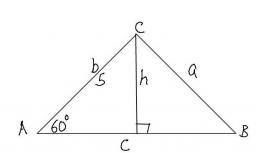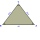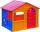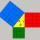# Base

Compute base of an isosceles triangle, with the arm a=20 cm and a height above the base h=10 cm.

b =  34.64 cm

### Step-by-step explanation:Did you find an error or inaccuracy? Feel free to write us. Thank you!Tips to related online calculators
Pythagorean theorem is the base for the right triangle calculator.

#### You need to know the following knowledge to solve this word math problem:

We encourage you to watch this tutorial video on this math problem:

## Related math problems and questions:

• ArmCalculate the length of the arm r of isosceles triangle ABC, with base |AB| = 14 cm and a height v=18 cm.
• Base of an isosceles triangleCalculate the size of the base of an isosceles triangle, the height is 5 cm and the length of the arm is 6.5 cm. What is the perimeter of this triangle?
• 3sides prismThe base of vertical prism is an isosceles triangle whose base is 10 cm and the arm is 13 cm long. Prism height is three times the height of base triangle. Calculate the surface area of the prism.
• Arm and baseThe isosceles triangle has a circumference of 46 cm. Calculate its area if the arm is 5 cm longer than the base.
• Isosceles triangleCalculate the size of the interior angles and the length of the base of the isosceles triangle if the length of the arm is 17 cm and the height to the base is 12 cm.
• CalculateCalculate the height to the base of the isosceles triangle ABC if the length of the base is c = 24cm and the arms have a length b = 13cm.
• CalculateCalculate the height of an isosceles triangle with base 37.8 mm long and an arm 23.1 mm long.
• Isosceles + prismCalculate the volume of the perpendicular prism if its height is 17.5 cm and the base is an isosceles triangle with a base length of 5.8 cm and an arm length of 3.7 cm
• Face of the houseHow tall is the gable of a house in the shape of an isosceles triangle with a base length of 8 meters and an arm 5 meters long?
• Right isoscelesCalculate area of the isosceles right triangle which perimeter is 26 cm.
• IS trapezoidCalculate the length of diagonal u and height v of isosceles trapezoid ABCD, whose bases have lengths a = |AB| = 37 cm, c = |CD| = 29 cm and legs b = d = |BC| = |AD| = 28 cm.
• Isosceles right triangleCalculate the area of an isosceles right triangle whose perimeter is 252 cm.
• Triangle eqCalculate accurate to hundredths cm height of an equilateral triangle with a side length 12 cm. Calculate also its perimeter and content area.
• Medians of isosceles triangleThe isosceles triangle has a base ABC |AB| = 16 cm and 10 cm long arm. What is the length of medians?
• Isosceles trapezoidFind the area of an isosceles trapezoid, if the bases are 12 cm and 20 cm, the length of the arm is 16 cm
• OPTWhat is the perimeter of a right triangle with the legs 14 cm and 21 cm long?
• Triangular prismCalculate the volume and surface of the triangular prism ABCDEF with base of a isosceles triangle. Base's height is 16 cm, leg 10 cm, base height vc = 6 cm. The prism height is 9 cm.